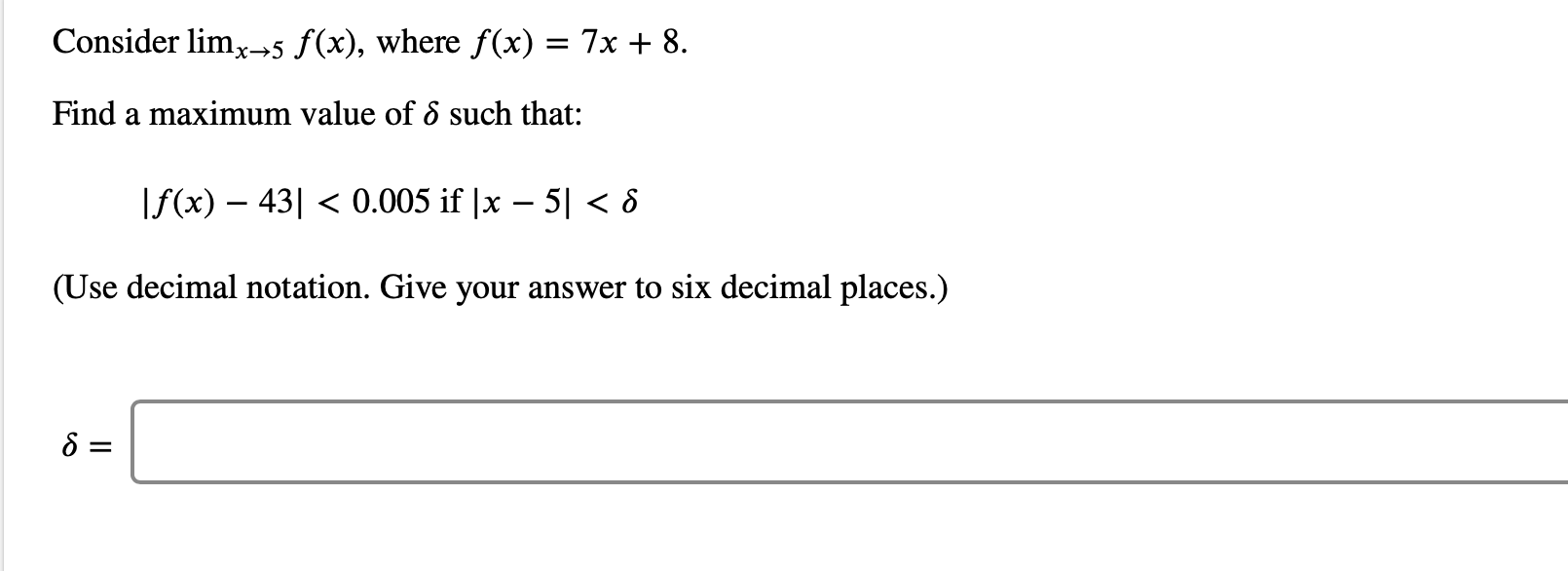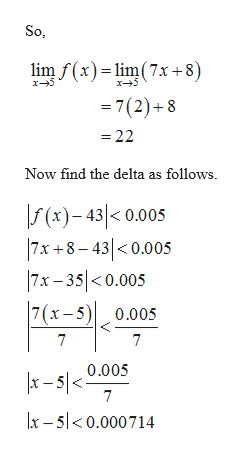Consider limx5 f(x), where f(x) = 7x + 8Find a maximum value of 8 such that:f(x) 430.005 if |x - 51 < 8(Use decimal notation. Give your answer to six decimal places.)

Questionhelp_outlineImage TranscriptioncloseConsider limx5 f(x), where f(x) = 7x + 8 Find a maximum value of 8 such that: f(x) 430.005 if |x - 51 < 8 (Use decimal notation. Give your answer to six decimal places.) fullscreen
Step 1

It is given that f(x) = 7x...help_outlineImage TranscriptioncloseSo lim f(x)lim(7x +8) x5 x5 =7(2)+8 22 Now find the delta as follows. (x)-43< 0.005 7x +8-430.005 7x- 35<0.005 7 (x-5)0.005 7 7 |x-5/<0.005 7 x-50.000714 fullscreen

Want to see the full answer?

See Solution

Want to see this answer and more?

Our solutions are written by experts, many with advanced degrees, and available 24/7

See Solution
Tagged in

Other